# 341 is the smallest composite number that gives a false positive for this Quick Prime Number Test

• 341 is a composite number.
• Prime factorization: 341 = 11 x 31
• The exponents in the prime factorization are 1 and 1. Adding one to each and multiplying we get (1 + 1)(1 + 1) = 2 x 2 = 4. Therefore 341 has exactly 4 factors.
• Factors of 341: 1, 11, 31, 341
• Factor pairs: 341 = 1 x 341 or 11 x 31
• 341 has no square factors that allow its square root to be simplified. √341 ≈ 18.466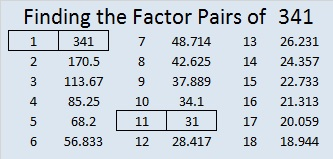341 is a composite number that sometimes acts like a prime number. To understand why, we need to understand a little bit about modular arithmetic:

When one number is divided by another, sometimes there is a remainder. Modular arithmetic is all about the remainders. We don’t care how many times one number divides into another number; we only care about the remainder.

Something very curious happens when the equation in the chart below is applied to a prime number greater than 2: The remainder is always 2. It really is! For example, 2^5 = 32 and 32 divided by 5 is 6R2. We say that 32 (mod 5) = 2 or 2^5 (mod 5) = 2 because 2 is the remainder. The fact that the remainder for prime numbers applied to this equation is always 2 is amazing, and can be a QUICK TEST to see if an odd number might be a PRIME NUMBER! If the remainder isn’t 2, that odd number is definitely NOT prime!

Passing the remainder test is a necessary but not a sufficient indicator that a number is prime: Even though 341 is not a prime number, the quantity 2^341 divided by 341 also has a remainder of 2. Since 341 = 11 x 31, but passes the remainder test, it is known as a pseudoprime number.  341 is the smallest composite number that passes this particular test, so 341 is an amazing number!

Also in the chart above, 2 is the most common remainder, followed by 8, then 32, then 128. All of those numbers are odd powers of 2. The even powers of 2 do not appear on the chart at all! That is a very curious phenomenon as well. (However, if x is an even number, it appears that y will usually be an even power of 2.)

Earlier mathematicians have written equivalent expressions and algorithms, but I prefer using “2^x (mod x)” because it takes very few keystrokes to enter into a calculator before hitting the equal sign:

Look at the image below.  It demonstrates that in prime-numbered rows, the numbers in that row can be divided evenly by that prime number (not counting the 1’s at the beginning and ending of each row).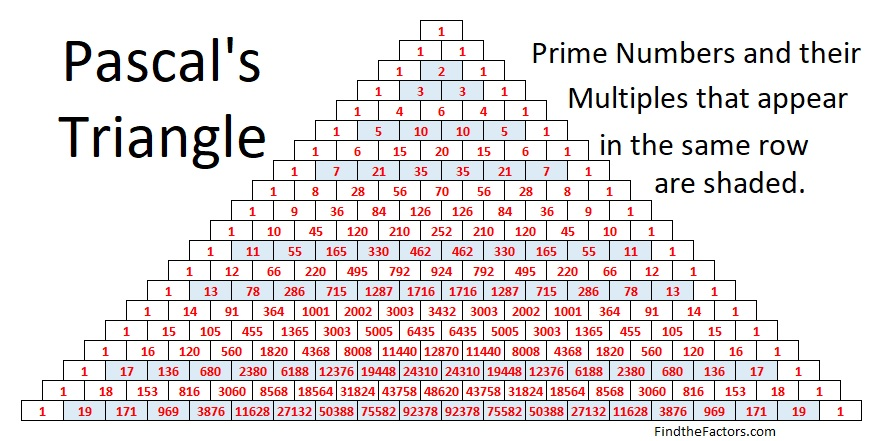• For a composite number, such as 15, at least one of the numbers in the row will NOT be divisible by that composite number. In row 15, 1 is the 0th term, 15 is the 1st term, 105 is the 2nd term, 455 is the 3rd term, and 1365 is the 4th term and so forth.
• Terms that are divisible by 15 are the 1st term (15), the 2nd term (105), the 4th term (1365), the 7th term (6435), the 8th term (6435), the 11th term (1365), and the 13th term (105). All of those term numbers, 1, 2, 4, 7, 8, 11, and 13, do NOT have factors in common with the number 15.
• The terms that are NOT divisible by 15 are the 3rd term (455), the 5th term (3003), the 6th term (5005), the 9th term (5005), the 10th term (3003), and the 12th term (455). All of those term numbers, 3, 5, 6, 9, 10, and 12, have at least one factor in common with the number 15.

If we could see the VERY large numbers for the 341st row, and if they weren’t expressed in Scientific Notation, we could note that the following terms would NOT be divisible by 341: terms numbered 11th, 22nd, 33rd, 44th, and so forth and the terms numbered 31st, 62nd, 93rd and so forth. However, if you add those terms together, that very large sum would be divisible by 341. That is so amazing, even though 341 is not prime!

This second image from Pascal’s Triangle demonstrates that the sum of the numbers in any row of Pascal’s triangle equals two raised to the second number in that row.
(There is no second number in the top row, so we could say that second number is zero, and 2º = 1.)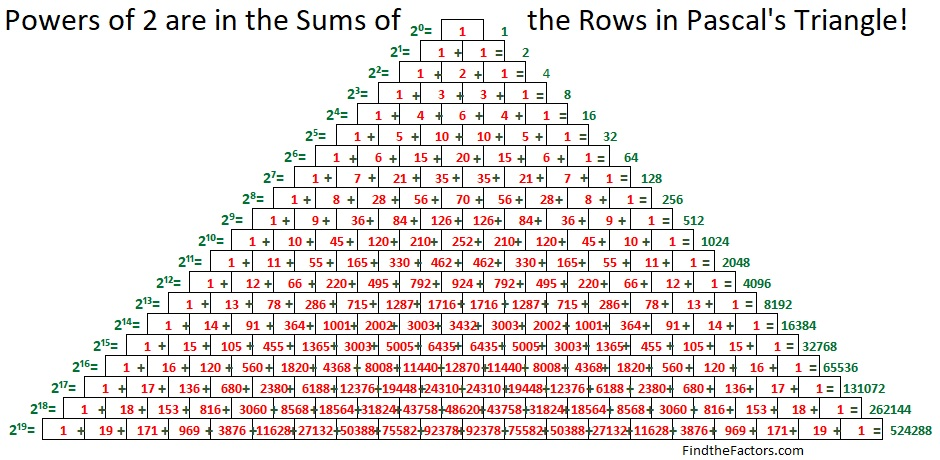These two images work together so that 2^p (mod p) will always be 2 for every prime number greater than 2 because every number in the prime-numbered rows can be evenly divided by that prime number. (Except the 1 at the beginning of the row and the 1 at the end of the row; Note 1 + 1 = 2)

Now that is why this amazing test for prime numbers works as well as it does while giving just a few false positives!

# 275 Are First-Graders Too Young to Learn About Odd and Even Numbers?

• 275 is a composite number.
• Prime factorization: 275 = 5 x 5 x 11, which can be written (5^2) x 11
• The exponents in the prime factorization are 2 and 1. Adding one to each and multiplying we get (2 + 1)(1 + 1) = 3 x 2  = 6. Therefore 275 has 6 factors.
• Factors of 275: 1, 5, 11, 25, 55, 275
• Factor pairs: 275 = 1 x 275, 5 x 55, or 11 x 25
• Taking the factor pair with the largest square number factor, we get √275 = (√11)(√25) = 5√11 ≈ 16.583Are six-year-olds too young to learn about odd and even numbers?

Paula Beardell Krieg gave me permission to use the pictures of this flexible number line she designed in this post:

I recently read a post at mathfour.com that discussed the “basic” concept of odd and even numbers and children’s ability to understand the difference. The article made me very curious so I talked briefly to 45 first grade students about even and odd numbers. What did I find out?

• Almost all of them had been introduced to the concept in kindergarten and knew that 1, 3, 5, 7, 9 are odd numbers while 2, 4, 6, 8, 10 are even.
• A few accelerated learning students were able to explain to me that the one’s digit of a number determines if the number is even or odd,
• But most of these first graders did not understand that fact because about a third of the students thought that 32 is odd!
• One little girl explained to me how odd and even numbers alternate. She said, “If 99 is even, then 100 will be odd.” She remembered that concept but didn’t understand it well enough to apply it to the example she gave!

Even though odd and even numbers may be a difficult concept to learn, teach the concept and use it anyway. In fact, talk about it to preschoolers while you put on their socks, shoes, or mittens.

Children learn to recite numbers in order before they learn how to count, and that helps them learn how to count and later how to add or subtract 1 from a number. I have tutored bewildered looking students who weren’t sure what to do with 8 + 1 = until I told them that 8 + 1 = means “what number comes right after 8 when you count?” Likewise, 8 – 1 = means “what number comes right before 8 when you count?” After hearing those questions, these students immediately knew the answer, and they didn’t count to find it.

Children who can quickly recite the odd numbers to 11 and the even numbers to 10 will have an easier time adding or subtracting two from a number. When they see 3 + 2 =, they can remember that 3 is odd and then ask themselves what odd number comes after 3. Likewise when they see 8 – 2, they can remember that 8 is even and recall that 6 is the even number right before 8.

The way I remember it, I was in second grade when I first was told that an even number plus an even number is even, an odd number plus an odd number is even, while an even number plus an odd number is odd. Any student learning to add or subtract would benefit from that tip.

Adding 3 to an odd number gives an even number, in fact, it’s the second even number after the original number. Adding 3 to an even number gives an odd number which is the second odd number after the original number. Subtracting 3 has the same rule, but substitute the word “before” for the word “after.”

Adding 4 to an odd number gives the second odd number after it while adding 4 to an even number gives the second even number after it. Subtracting 4 has a similar rule.

Adding 3 or 4 will mean additionally memorizing that 12 and 14 are even and 13 is odd, but that will be all a first grader needs to know about odd and even numbers. Later these two categories of numbers will be useful throughout their lives for many, many reasons.

What are some ways to help children to memorize odd and even numbers? Paula Beardell Krieg has designed the most captivating number line in the world.

It can easily go from looking like this:

to looking like this:

The transformation can be done by a child or an adult. This number line that is made with envelopes is pretty enough to hang on a classroom wall, but it can fold up like a book, or be played with and changed so that real learning can take place. Paula Beardell Krieg shows several uses of it in her post, the-flux-capacity-of-an-artful-number-line, and promises to give directions on how to make one soon!

Try these rhymes: 0, 2, 4, 6, 8; Being EVEN is just great! 1, 3, 5, 7, 9; Being ODD is just fine!

Smartfirstgraders.com has several activities and rhymes to help students memorize the odd and even numbers.

• Finally, if you clap when you say ODD, you will clap one time. 1 is an odd number.
• If you clap when you say EVEN, you will clap two times, 2 is even.
• And as mathfour.com pointed out in more detail then I’m showing here: ODD has 3 letters, and 3 is odd.
• Also EVEN has 4 letters to help us remember that 4 is even.

# 21 Factors of the Year 2013 and 2014

21 is a composite number. 21 = 1 x 21 or 3 x 7. Factors of 21: 1, 3, 7, 21. Prime factorization: 21 = 3 x 7.When 21 is a clue in the FIND THE FACTORS puzzles, use 3 x 7.

Scroll down the page to find factoring information about 2013 and 2014.Near the end of each year movie critics make lists of the ten best movies and the ten worse movies of the year. News agencies list the ten most significant news stories. Time magazine lists the ten most influential people of the year. The music industry lists the top ten songs of the year. As 2013 draws to a close, it is most appropriate for me to review the factors of the year.

2013 had exactly 8 positive factors. These factors were 1, 3, 11, 33, 61, 183, 671, and 2013.

There is no room for argument. I am absolutely certain this list is complete. No one will make any comments disagreeing with me, calling me names, or asking how I could have left Two or Five or Seven off the list. Also no one will wonder why I would include forgettable 671 on the list. Do the Math. 671 was clearly a factor in 2013. Three of the factors of 2013 were also prime factors. They were 3, 11, and 61. This graphic clearly shows those prime factors.2013 also had 8 negative factors. The first negative factor on the list is no surprise: Minus One. Year in and year out we can count on Minus One being a negative factor. Some other factors were just as negative in 2013, namely -3, -11, -33, – 61, -183, -671, and -2013. Of course, many of those factors were so obscure that most people never gave them a second thought all year long. Again I expect no arguments or negative comments on these selections. Anyone who knows anything about factors will have to agree with this list.

Even though 2014 hasn’t even started, I am going to predict the factors of 2014, and I am absolutely positive that my predictions will be 100% correct. You will not even have to wait until the end of 2014 to verify my accuracy.

The positive factors of 2014 will be (drum roll) 1, 2, 19, 38, 53, 106,1007, and 2014.

Most people expect the number One to be a positive factor every single year, and it will not let us down in 2014. The number Two has a reputation of being a factor only about half the time. Since she was not a factor at all in 2013, I am confident that she will get her act together again in 2014 and become a factor once more. All the other factors I’ve listed have not been factors for a very long time, and each one of them is due to make a difference over and over again in 2014 until they have nothing leftover. I predict that 2014 will have three prime factors, namely 2, 19, and 53, as illustrated in the following graphic.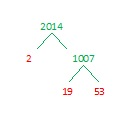How can I make such accurate assessments and spot on predictions? I will tell you: I work with factors almost every single day, and I’ve spent years observing them. Every time I have been given an assignment to become acquainted with them, I have approached that assignment with enthusiasm and determination.

Regardless of my astounding record, YOU can become just as much an expert as I am with just a little bit of knowledge and effort. You may discover, as I have, that factoring can be great fun. Here are a couple of logic puzzles that require factoring to solve:

All you have to do to solve one of the puzzles is write the numbers 1 – 12 in the top row and again in the first column so that those numbers are the factors of the given clues. Each puzzle has only one solution.

At the top of this post is a page titled How to Find the Factors, and it gives hints to solve the puzzles.   Click 12 Factors 2013-12-30 to find a printable version of these and a few other puzzles as well as the solutions for last week’s puzzles. Excel or comparable spreadsheet program is needed to open the file.

Have a great 2014 and happy factoring!

Related Articles

# 6 A Piece of Cake

### Birthday Puzzles for My Daughter:

Happy birthday, Kathy! I hope your day is wonderful. You have grown into a beautiful, talented, prayerful, intelligent, hard-working, and loving young woman.  I am grateful you are my daughter.  So for your birthday today and for this blog, I’ve created three special puzzles: the first is a birthday cake to celebrate your happy day. To highlight your love of music, the second puzzle is a quarter note. The third puzzle is either a violin, a guitar, or a ukulele, you decide. I love listening as you sing or as you play any of those instruments or the piano. Today for your birthday I will also cut down a tree and make yet another cake with two birthday candles on top in this blog post.  So have a fun birthday, today.  I love you.Click 12 Factors 2013-11-14 for more puzzles.### Factor Trees vs. Factor Cakes:

What did I mean by cutting down a tree and making yet another cake? Today I will discuss two methods for finding the prime factors of a whole number. One method is making a factor tree and the other is the cake method. To factor a number means to write it as the product of two or more factors. When those two or more factors are all prime factors, it is called a prime factorization of the number. A composite number always has more than two factors. A prime number always has exactly 2 factors, 1 and itself. (ZERO and ONE are neither prime or composite numbers.) Usually, to find the prime factors of a number, a person will usually make a factor tree. The following example shows how this is done:From this example, you can certainly understand why this algorithm is called a factor tree.  It looks exactly like a perfectly-shaped evergreen tree.  The problem is that a factor tree doesn’t always look so neat and trim.  Here is a factor tree that even Charlie Brown wouldn’t choose:720 isn’t even that big of a number, but gathering all of the prime numbers from the factor tree and putting them in numerical order would be like picking up a bunch of scattered leaves. It would be like doing . . . yard work.  Imagine if you had a number that had many more factors. If one or two of the factors gets lost in the mess, your answer wouldn’t be correct. Notice that some of the prime factors of 720 (2,2,2,2,3,3,5) are not as easy to see as others on the factor tree.  That is why I want to chop down that tree. Even if you like to do yard work, do you really want to deal with that big of a mess, . . . especially when you can have cake instead?  Look, the cake method is so much more pleasing to the eye, and it is simply an extension of the very familiar division algorithm:With the cake method, the more factors you have, the bigger the cake will be, but it will always be neatly organized with all the factors on the outside of the cake.  And if the largest prime factor of your given number is eleven, you will also have two candles on top of your cake!  I find using the cake method to be much less confusing than using a factor tree.  Yes, finding prime factors can actually be a piece of cake. The only disadvantage to the cake method is that since you work from the bottom up you have to leave enough space for the cake to rise.

Still, in spite of my opinion, it is best to use whichever method you are more comfortable with.

Now if your appetite for cake has not been satisfied, click on one of the links below for a nice variety of cakes shared by other bloggers.

### Factors of the Number 6:6 is a composite number. 6 = 1 x 6 or 2 x 3. Factors of 6: 1, 2, 3, 6. Prime factorization: 2 x 3.When 6 is a clue in the FIND THE FACTORS  puzzle, the pair that will work for that particular puzzle might be 1 x 6, or it might be 2 x 3.

### A Sum-Difference Puzzle Featuring the Number 6 and its Factors:

Look at the factor pair puzzle above. Perhaps you will notice that
2 + 3 = 5 and 6 – 1 = 5.
Those are the facts you need to complete the Sum-Difference puzzle below.# 5 Easy as 1-2-3

• 5 is a prime number.
• Prime factorization: 5 is prime.
• The exponent of prime number 5 is 1. Adding 1 to that exponent we get (1 + 1) = 2. Therefore 5 has exactly 2 factors.
• Factors of 5: 1, 5
• Factor pairs: 5 = 1 x 5
• 5 has no square factors that allow its square root to be simplified. √5 ≈ 2.236.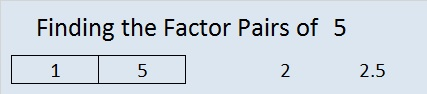How do we know that 5 is a prime number? If 5 were not a prime number, then it would be divisible by at least one prime number less than or equal to √5 ≈ 2.2. Since 5 cannot be divided evenly by 2, we know that 5 is a prime number.

5 is the only number that is the sum of ALL the prime numbers less than itself.

2² + 1² = 5 and 3² + 4² = 5² so 5 is the smallest Pythagorean triple hypotenuse.

When 5 is a clue in a FIND THE FACTORS puzzle, use 1 for one of the factors and 5 for the other.

Being able to identify factors of a whole number is a very important skill in mathematics.  It is a skill that is commonly used in many areas of mathematics ranging from reducing fractions to solving differential equations.  The Find the Factors puzzles can help make that skill second nature.Click 10 Factors 2013-11-11 for more puzzles.

To solve the puzzles, we are only interested in the limited set of factors that are represented in the following table: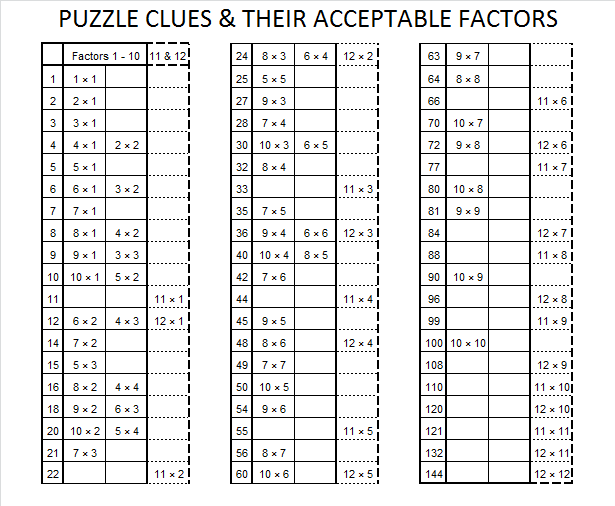What about all the other factors of these numbers?  And what about all the other whole numbers not on the chart?  How do you find ALL of the factors of a given whole number?  For example, suppose you were asked to find all of the factors of 435.  Some people might notice right away that it is divisible by 5 because its last digit is 5.  While that is true, beginning with 5 is not the best place to start because there is an advantage in considering all possible factors in an organized way.  When you are asked to find ALL of the factors of any number, starting at 1 will make finding all of the factors as easy as 1-2-3. So what are the factors of 435?  Using a calculator, I notice that the square root of 435 is about 20.85.  That means I can find absolutely all of the factors of 435 by considering as  divisors just the whole numbers from 1 to 20!  Each factor will have a partner that is greater than 20 but will be found at the same time with these few short calculations. To demonstrate my thinking process, I will put each possible factor from 1 to 20 in a chart and write my thoughts as I consider each one.As you may notice, once a possible factor is eliminated, it is not necessary to do any actual division by ANY of the multiples of that number. (4, 6, 8, 10, 12, 14, 16, 18, and 20 are all multiples of 2, which was not a factor, so I didn’t actually divide 435 by any of those multiples.)

As I carefully consider each possible factor, I only WRITE DOWN a number if it is an actual factor.  Therefore, with only a little bit of effort I would list ALL of the factors of 435 in one tidy list: 1 x 435, 3 x 145, 5 x 87, 15 x 29.

See, it was as easy as 1-2-3!  Now let’s find all of the factors of 144.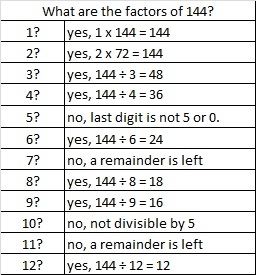Even though 144 is less than 435, it has more factors. One of its factors is paired with itself because the square root of 144  is 12.  That fact is also the signal that we can stop looking for more factors, and we can list all the factors of 144 on the following chart:There are 8 multiplication facts that produce 144, but 12 x 12 = 144 is the only fact we consider when solving a Find the Factors 1-12 puzzle with 144 as one of the clues. In every other case one of the pair of numbers in the multiplication fact will be greater than 12 and not eligible to be written in the factor row or factor column. However in solving mathematical problems, any of the factors of a whole number could be the star of the show. Knowing how to find those factors is indeed an important skill and is as easy as 1-2-3.